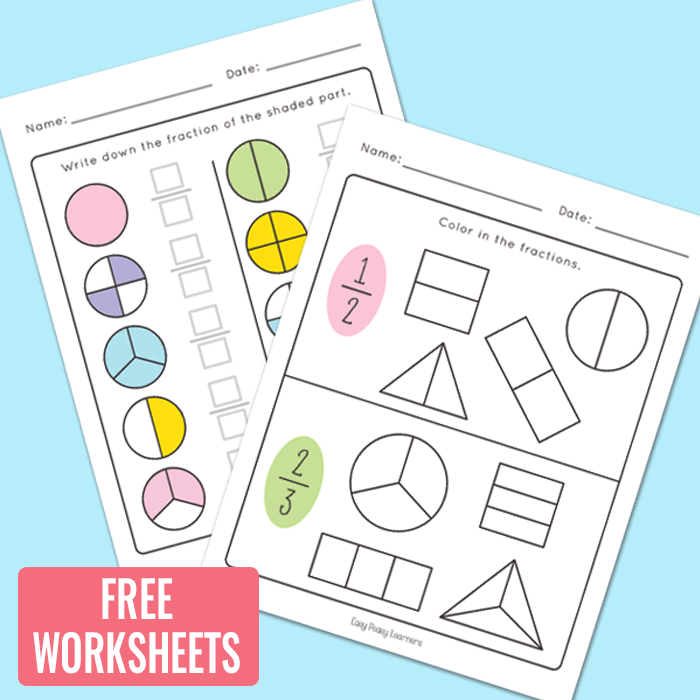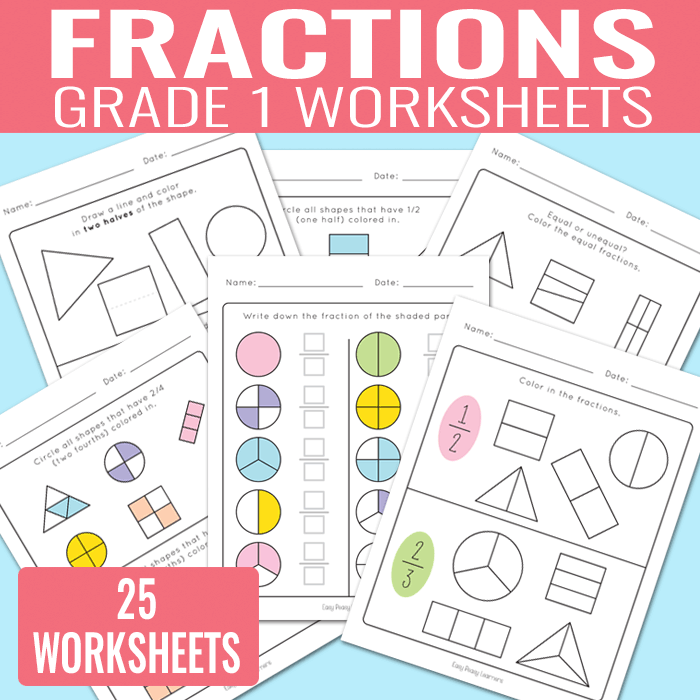# Fractions Worksheets for Grade 1

It’s time to practice fractions with this set of fractions worksheets for grade 1.

From coloring in to writing down fractions, these worksheets are great both for learning and fraction revision (test prep or homework).This printable set includes 25 different worksheets (+ 5 worksheets with “quarters” instead of fourths)

What you will get in this printable worksheet set;

• 5 color the fractions worksheets. Students look at the numeric fractions and color in the given fraction on different shapes.
• 5 write the fractions. Students look at the shapes and the parts that are colored in. They are asked to write down the fractions.
• 5 circle the shapes fractions worksheets. Students are given a fraction and have to circle all the shapes in a group that represent that fraction.
• 5 equal or unequal fractions. Shapes are divided in equal and unequal parts. Students are asked to color in the shapes that have equal fractions.
• 5 draw a line and color. Students are given a fraction and are asked to split the shapes into parts and color in the given fractions.

These are great for student homework and test prep.

You can get the whole set at Teachers Pay Teachers where you can also preview the whole set.

Educators can laminate these worksheets to be used with dry erase markers or page protectors. This set comes in full color but is optimised for black and white printing.Want to try out these worksheets? You can grab yourself a set